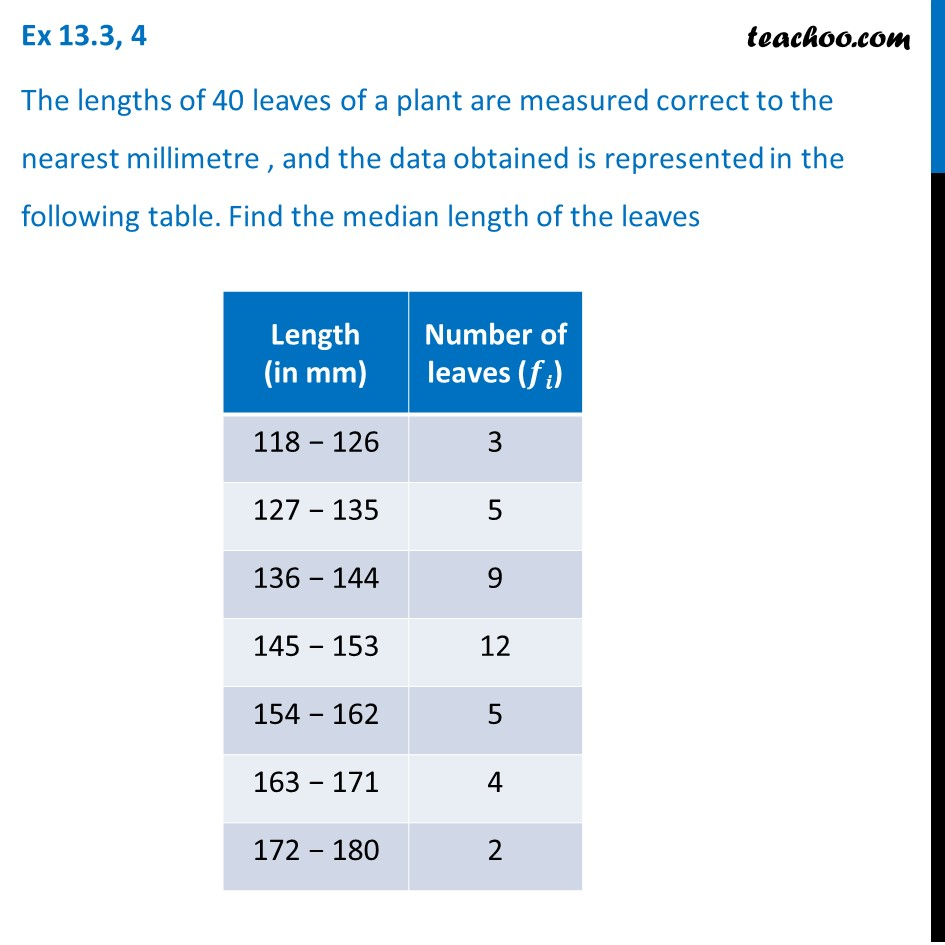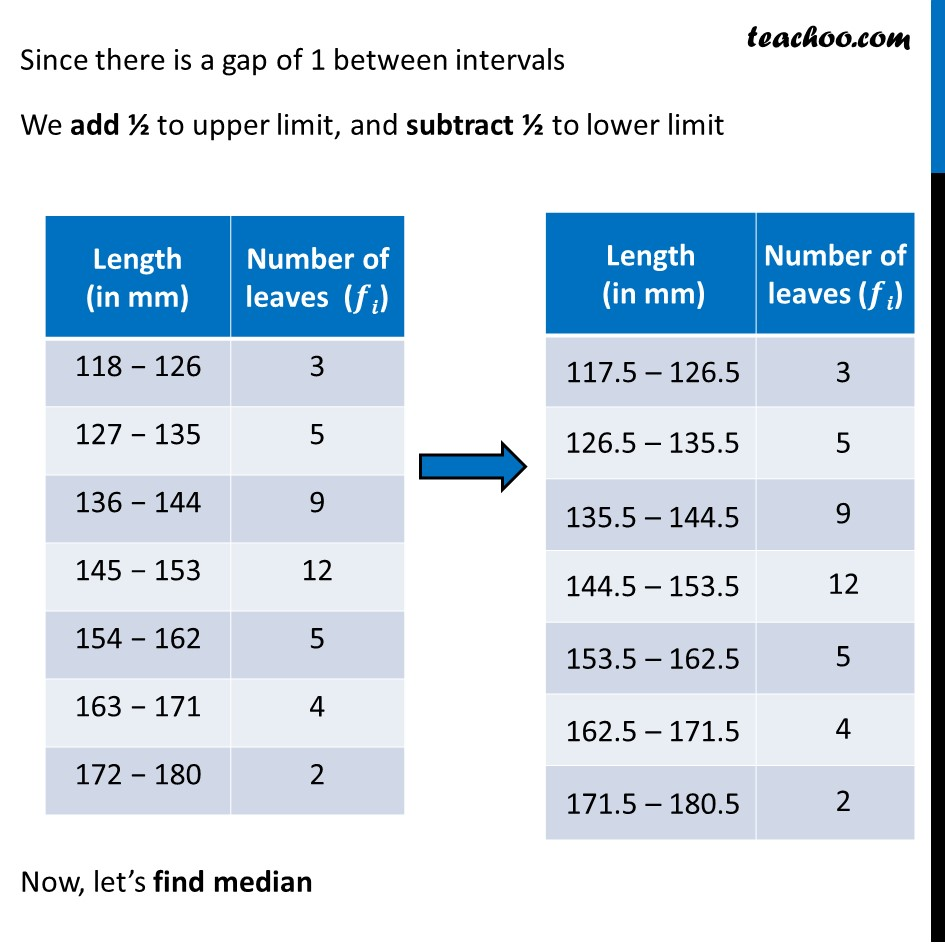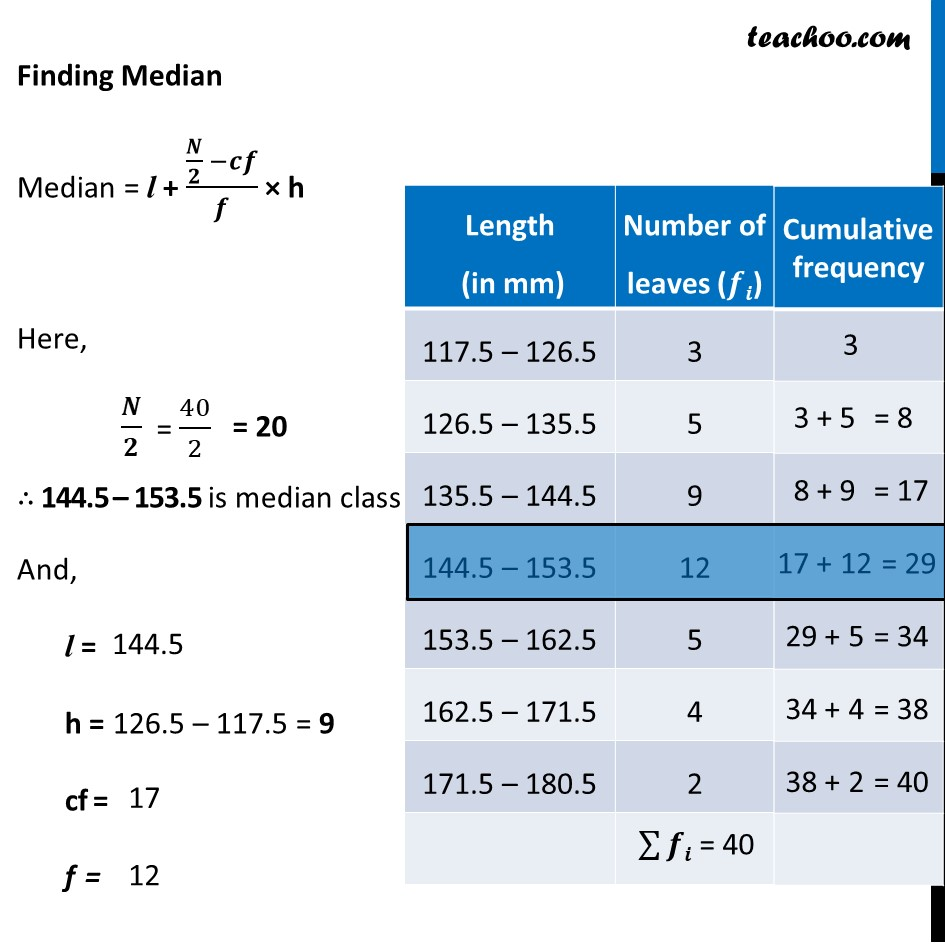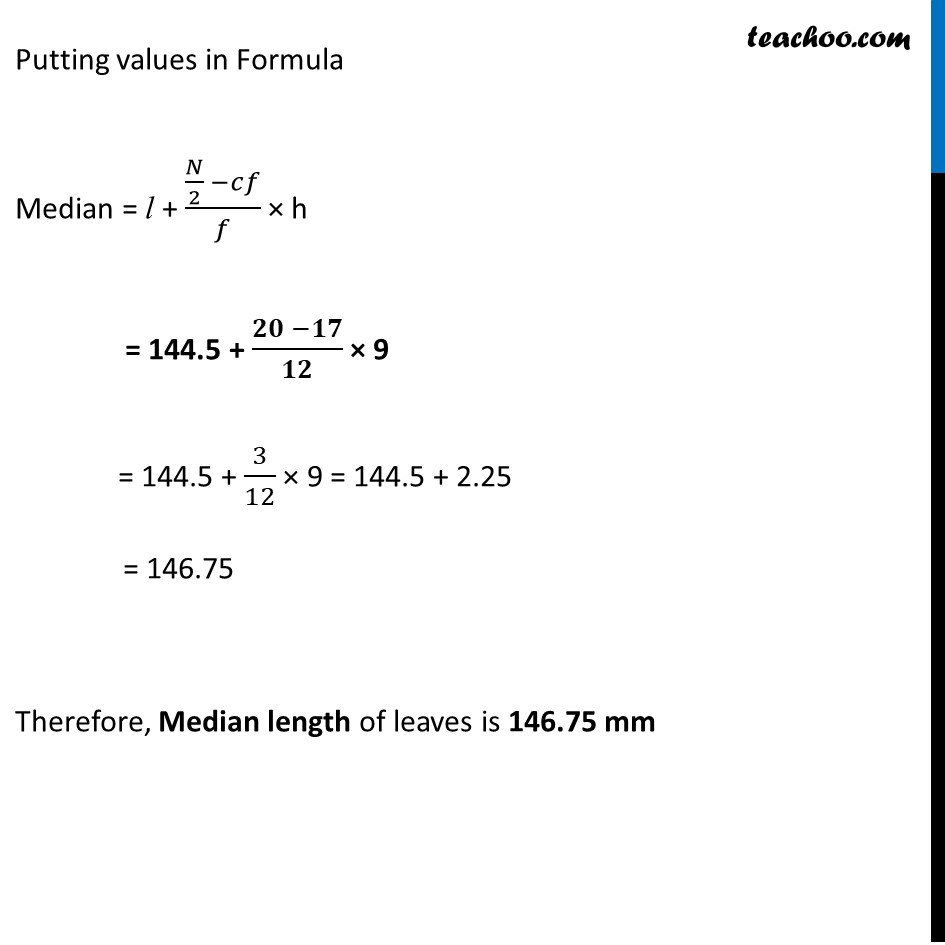Chapter 14 Class 10 Statistics

Class 10
Important Questions for Exam - Class 10Learn in your speed, with individual attention - Teachoo Maths 1-on-1 Class

### Transcript

Ex 13.3, 4 The lengths of 40 leaves of a plant are measured correct to the nearest millimetre , and the data obtained is represented in the following table. Find the median length of the leaves Since there is a gap of 1 between intervals We add ½ to upper limit, and subtract ½ to lower limit Now, let’s find median Finding Median Median = l + (𝑵/𝟐 −𝒄𝒇)/𝒇 × h Here, 𝑵/𝟐 ∴ 144.5 – 153.5 is median class And, l = h = cf = f = Putting values in Formula Median = l + (𝑁/2 −𝑐𝑓)/𝑓 × h = 144.5 + (𝟐𝟎 −𝟏𝟕)/𝟏𝟐 × 9 = 144.5 + 3/12 × 9 = 144.5 + 2.25 = 146.75 Therefore, ﻿Median length of leaves is 146.75 mm